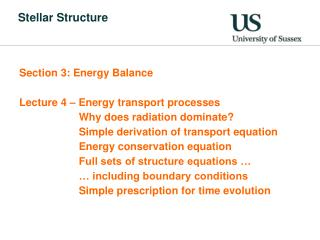Download PresentationStellar Structure

# Stellar Structure - PowerPoint PPT Presentation

Stellar Structure. Section 3: Energy Balance Lecture 4 – Energy transport processes Why does radiation dominate? Simple derivation of transport equation Energy conservation equation Full sets of structure equations … … including boundary conditionsI am the owner, or an agent authorized to act on behalf of the owner, of the copyrighted work described.
Download Presentation## Stellar Structure

Download Policy: Content on the Website is provided to you AS IS for your information and personal use and may not be sold / licensed / shared on other websites without getting consent from its author.While downloading, if for some reason you are not able to download a presentation, the publisher may have deleted the file from their server.

- - - - - - - - - - - - - - - - - - - - - - - - - - E N D - - - - - - - - - - - - - - - - - - - - - - - - - -
Presentation Transcript
1. Stellar Structure Section 3: Energy Balance Lecture 4 – Energy transport processes Why does radiation dominate? Simple derivation of transport equation Energy conservation equation Full sets of structure equations … … including boundary conditions Simple prescription for time evolution

2. Energy transport – from central energy source to surface emission • Radiation – energy carried by photons • Conduction – energy carried by electrons (mainly) • Convection – energy carried by large-scale gas motions Convection: discussed later (Section 4) Radiation, conduction similar, so can be treated similarly, using a conduction coefficientλ(see blackboard) Which carries more energy? Normally (see blackboard) gas particles possess more energy than photons – but photons travel much farther: ℓphoton ≈ 105 ℓelectron . Exception: high density stars (e.g. WDs) (Pauli exclusion principle)

3. Energy carried by radiation:approximate argument (see blackboard) ℓ = photon mean free path ℓ = r (T+T)4  = opacity (defined below) T T + T T4 r r + r  = cross-sectional area for absorption (per unit volume)

4. Energy carried by radiation • Approximate argument gives: • Precise argument (see Handout) gives: • Hence (see blackboard): (3.23) • Finally, energy conservation in a spherical shell (see blackboard) gives: (3.24)

5. The four differential equations of stellar structure Assuming (i) steady state (ii) all energy carried by radiation: 4 equations 7 variables

6. Three relations to close the system Assuming that conditions in stellar interiors are close to thermodynamic equilibrium: P = P(, T, composition)  = (, T, composition) ε = ε(, T, composition). Using previous explicit expression for pressure: we also need  = (, T, composition).

7. Differential equations in terms of mass as independent variable Surface best defined by M = Ms, so use mass as variable: (dividing (2.1) by (2.2)) (3.29) (3.30) (3.31) (3.32) Then central boundary conditions are: r = L = 0 at M = 0. (3.33)

8. Surface boundary conditionsVogt-Russell “theorem” • Surface boundary conditions? • A useful approximation is:  = T = 0 at M = Ms . (3.34) • Actually, Ts ≠ 0 – and is one of the unknowns. But can find effective temperature from Ls4πRs2Teff4 since Ls, Rs emerge from solution, and assume Ts ≈ Teff. • Vogt-Russell “theorem”: 7 equations of stellar structure, plus 4 boundary conditions, completely determine the structure of a star of given mass and composition. Unique for main-sequence stars, but not for evolved stars – may also depend on history.

9. Approximate treatment of stellar evolution • Simple treatment uses sequence of static models, differing only in composition: • Build initial model, with composition known as function of mass. • Assume all energy release from nuclear reactions. • Calculate resultant changes in composition (schematic): . (3.35) • Integrate (3.35) for timestep Δt, starting from composition of previous model. • Construct sequence of static models of gradually changing composition and structure.